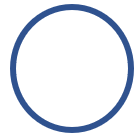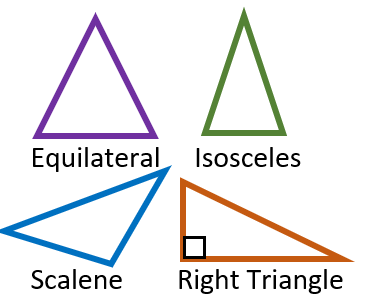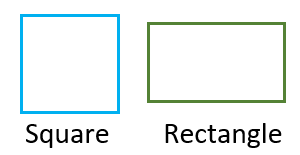# Math Worksheets Land

Math Worksheets For All Ages

# Math Worksheets Land

Math Worksheets For All Ages

Home > Topics >

# Geometric Shapes Worksheets

Before children learn about advanced and complicated algebraic concepts, they must have a firm grip on the basic geometric shapes. When we understand basic outlines of these models, we can begin to grasp what is going on inside them as well. Shape recognition and identification is the start of geometry. Take a look at our geometry section for worksheets that actually use the calculation of shapes. Students need to learn how to identify all these forms. We start by identification these forms based on the number of sides that they have. We then progress to counting the number of corners and vertices. The last step is understanding the measures of their interior angles and the measure of the sum of all of them. I also like my students to be able to know the various delineations of shapes such as unique forms of triangles and quadrilaterals. These topics below will start you students in the right direction with classifying, scaling, and even drawing all these different forms.

### What are Geometric Shapes?

Figures closed by a definite boundary formed by specific curves, points and lines are defined as geometric shapes. All of us are familiar with the common geometric shapes like a rectangle, circle, triangle and square. If we have a solid understanding of them it comes in very handy during construction projects. As you go through life and encounter people that are in the construction industry you will quickly realize that they understand just about every property that these shapes have in both two and three dimensions.

### How Do They Get Their Names?

They get their names based on a variety of factors. It normally begins with number of sides that they have. It can also be based on the angles found with the shape or if the sides are equal in length. We will explore the most commonly found basic two-dimensional geometric shapes. Each form has its own unique properties.Circle - A circle is a geometric shape with no straight lines. It is formed by combining and connecting curves together. A circle has no angles, corners, or vertices. In real life you will find that cookies, pizzas, and wheels all share this shape. Most dinner plates and analog clocks do too.

Triangle - A triangle comprises of three-line segments connected. As a result, they have 3 edges and vertices. The measure of the sum of all three interior angles is 180°. Unlike a square or rectangle, angles are of different measurements in a triangle. A triangle does not always have the right angle. The type of angle found within a triangle defines the kind of triangle. For example, if a triangle has a right angle, it will be named as a right-angled triangle. If all the angles are equal it is called an equilateral. If two sides and angles are equal it is called isosceles. If all three angles and sides are different it is called scalene. You find triangles in daily life in the form of pizza slices, yield traffic signs, and pretty much when ever you make a diagonal cut on paper or rectangular bread.Square - A four-sided geometric shape created by joining four-line segments is known as a square. The four-line segments of a square are of equal lengths and form four right angles. They have four sides and four vertices. Square is commonly the shape of floor and ceiling tiles. If you play checkers or chess this is the form of the board itself.

Rectangle - A rectangle is like a square, and it is also formed by combining four-line segments. The only difference between square and rectangle is that in a rectangle, the four-line segments are not if equal lengths. It has two-line segments longer than the other two-line segments. Also, the four corners of a rectangle form the four right angles. This is the form of most text books, smart phones, and picture frames.These are the core geometric shapes that you will work with as you move on to working with more sides and angles, there will be new names. This does give you a good foundation to from.

Unlock all the answers, worksheets, homework, tests and more!
Save Tons of Time! Make My Life Easier Now

## Thanks and Don't Forget To Tell Your Friends!

I would appreciate everyone letting me know if you find any errors. I'm getting a little older these days and my eyes are going. Please contact me, to let me know. I'll fix it ASAP.